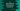# C program to print the ASCII values of all lowercase characters# Introduction to ASCII :

ASCII or American Standard Code for Information Interchange is used to represent a letter, number and other characters in integer form. There are 256 values starting from 0 to 255. In this C tutorial, we will learn how to print the ASCII values of all lowercase characters using a C program.

## C program to print ASCII of all lowercase characters :

``````#include <stdio.h>
int main()
{
//1
int start = 'a';
//2
while (start <= 'z')
{
//3
printf("%c : %d\n", start, start);
//4
start++;
}
return 0;
}``````

### Output :

It will print all characters and their ASCII characters from a to z.

``````a : 97
b : 98
c : 99
d : 100
e : 101
f : 102
g : 103
h : 104
i : 105
j : 106
k : 107
l : 108
m : 109
n : 110
o : 111
p : 112
q : 113
r : 114
s : 115
t : 116
u : 117
v : 118
w : 119
x : 120
y : 121
z : 122``````

### Explanation :

The commented numbers in the above program denote the step numbers below :

1. create one integer variable start and assign ‘a’ to it.
2. We are printing the values using a while loop. This loop will run until the value of start is less than or equal to ‘z’.
3. Inside the loop, print out the character and its ASCII value. You can see that for printing the character, we are using %c and for ASCII value, we are using %d.
4. Inside the loop, on each iteration, after the character and ASCII value is printed, we are incrementing the value of start by one. So, next time it will print the ASCII value of the next character. The loop will exit when the current character becomes ‘z’.

### Conclusion :

In this tutorial, we have learned how to print the ASCII value of a specific character in c. We have used one while loop to print all characters and their ASCII values in a range. Instead of a while loop, we can also use one for loop to do the same. Try to run the program and if you have any queries, drop one comment below.